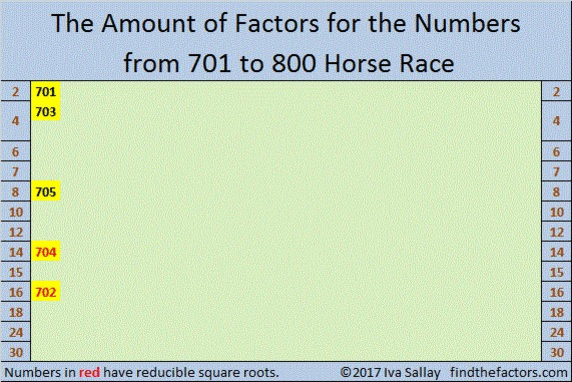# 800 Which Pony Will Take Second Place?

Every 100 posts I summarize the amount of factors of the previous 100 numbers.

MANY of the numbers from 701 to 800 have FOUR factors, and any other number-of-factors doesn’t even come close. For this Horse Race, SECOND place is much more interesting as there are several lead changes. I’ve shorten the track so the second place number-of-factors can reach the finish line.

So go ahead, pick the number-of-factors pony you think will come in SECOND place. Your best bets are 2, 6, 8, 12, 16 OR the second row of 4 factors!

Make your selection, then click on the graphic below to see how your pony does!Now let me tell you a little bit about the number 800.• 800 is a composite number.
• Prime factorization: 800 = 2 x 2 x 2 x 2 x 2 x 5 x 5, which can be written 800 = (2^5) x (5^2)
• The exponents in the prime factorization are 5 and 2. Adding one to each and multiplying we get (5 + 1)(2 + 1) = 6 x 3 = 18. Therefore 800 has exactly 18 factors.
• Factors of 800: 1, 2, 4, 5, 8, 10, 16, 20, 25, 32, 40, 50, 80, 100, 160, 200, 400, 800
• Factor pairs: 800 = 1 x 800, 2 x 400, 4 x 200, 5 x 160, 8 x 100, 10 x 80, 16 x 50, 20 x 40 or 25 x 32
• Taking the factor pair with the largest square number factor, we get √800 = (√400)(√2) = 20√2 ≈ 28.28427.800 is the sum of four consecutive primes:

• 193 + 197 + 199 + 211 = 800

800 is a palindrome in three different bases.

• 2222 BASE 7 because 2(7^3) + 2(49) + 2(7) + 2(1) = 800 (This fact also means that x^3 + x² + x – 399 can be divided evenly by x – 7.)
• 242 BASE 19 because 2(19²) + 4(19) + 2(1) = 800
• PP BASE 31 (P is 25 base 10) because 25(31) + 25(1) = 800

800 is the sum of two squares two different ways:

• 28² + 4² = 800
• 20² + 20² = 800

That being true, it follows that 800 is the hypotenuse of two Pythagorean triples:

• 480-640-800 which is 160 times 3-4-5
• 224-768-800 which is 32 times 7-24-25

800 is also the sum of three squares:

• 20² + 16² + 12² = 800

This chart summarizes the number of factors for the first 800 numbers and indicates that 39% of those numbers have square roots that can be simplified (reduced).In case you didn’t click on the Horse Race image before, here it is, no clicking required:make science GIFs like this at MakeaGif